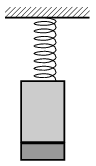Mathematical and Physical Journal
for High Schools
Issued by the MATFUND Foundation
 Already signed up? New to KöMaL?

#Problem P. 4557. (September 2013)

P. 4557. A 0.6-kg iron cylinder is attached to the end of the spring shown in the figure, and a disc shape magnet of mass 0.2 kg sticks to the bottom of the iron cylinder. Initially the spring, the spring constant of which is 64 N/m, is unstretched, and then the system is released. During the oscillation the magnet does not fall off.

a) What is the period of the oscillation?

b) What is the greatest speed of the magnet?

c) What is the minimum force between the magnet and the iron cylinder?(4 pont)

Deadline expired on October 10, 2013.

Sorry, the solution is available only in Hungarian. Google translation

Megoldásvázlat. $\displaystyle a)$ 0,7 s.

$\displaystyle b)$ 1,1 m/s.

$\displaystyle c)$ 3,9 N.

### Statistics:

 113 students sent a solution. 4 points: 52 students. 3 points: 42 students. 2 points: 7 students. 1 point: 11 students. 0 point: 1 student.

Problems in Physics of KöMaL, September 2013